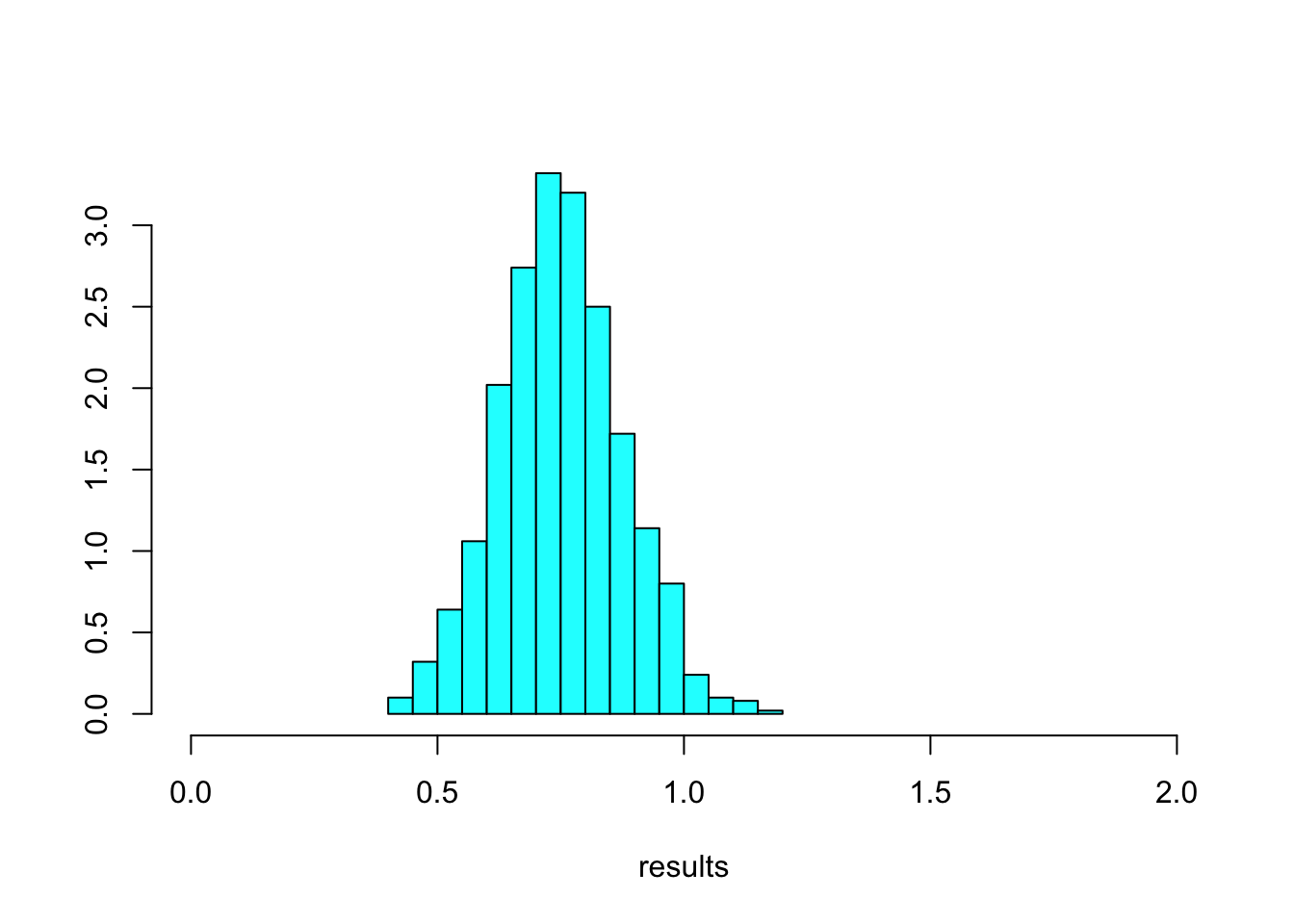## This simulation programme generates an N x 4 spreadsheet
## containing the numbers -1 and +1, and two lists of binary
## settings for Alice and Bob (setting 1 and 2 corresponding to
## outcomes of 2 N fair coin tosses) such that the
## quantum mechanical prediction of the value of S for
## the CHSH measurements on the singlet state is approximately
## reproduced, when the settings are used to select outcomes
## and compute the experimentally observed CHSH quantity.

## The spreadsheet contains a set of N counterfactual outcomes
## for each of the N iterations of the experiment, ie the outcomes
## which would be observed if either of Alice's two settings and
## either of Bob's two settings had been chosen.

## Combined with the two sets of actually chosen settings we
## obtain the actual experimental record: for each iteration,
## a setting and an outcome for Alice and for Bob each.

## After this, we compute four observed correlations
## and the CHSH quantity S.

## The N x 4 spreadsheet of sets of counterfactual outcomes,
## and the N x 4 spreadsheet with the actually chosen settings
## and resulting experimental record, are both output as csv files.

## A little simulation experiment shows that the observed result was
## actually extremely lucky. Repeating 1000 times with new random
## settings, we never even see one violation of CHSH. However, with
## a different random seed, it could occasionally happen ...
## which is the point of the whole thing here.

## Reference:

## Gill, Richard D. "Statistics, Causality and Bell’s Theorem".
## Statist. Sci. 29 (2014), no. 4, 512--528. doi:10.1214/14-STS490.
## http://projecteuclid.org/euclid.ss/1421330545

## http://arxiv.org/abs/1207.5103
## https://pubpeer.com/publications/D985B475C637F666CC1D3E3A314522

## Files (one R script; two output files produced by the script):

set.seed(1234)

N <- 800
S <- 2 * sqrt(2)
rho <- S / 4
quantumProbs <- c((1 + rho)/2, (1 - rho)/2, (1 - rho)/2, (1 + rho)/2)

settingsAlice <- sample(c(1, 2), N, replace = TRUE)
settingsBob <- sample(c(1, 2), N, replace = TRUE)

spreadsheet <- matrix(sample(c(-1, +1), N * 4, replace = TRUE), N, 4)

quantumOutcomes <- sample(4, N, quantumProbs, replace = TRUE)
quantumAlice <- 2 * (quantumOutcomes %in% c(1, 2)) - 1
quantumAlice[settingsAlice == 2 & settingsBob == 2] <-
- quantumAlice[settingsAlice == 2 & settingsBob == 2]
quantumBob <- 2 * (quantumOutcomes %in% c(1, 3)) - 1

spreadsheet[settingsAlice == 1, 1] <- quantumAlice[settingsAlice == 1]
spreadsheet[settingsAlice == 2, 2] <- quantumAlice[settingsAlice == 2]
spreadsheet[settingsBob == 1, 3] <- quantumBob[settingsBob == 1]
spreadsheet[settingsBob == 2, 4] <- quantumBob[settingsBob == 2]

colnames(spreadsheet) <- c("A", "A'", "B", "B'")
head(spreadsheet)
##       A A'  B B'
## [1,]  1 -1  1 -1
## [2,] -1 -1  1  1
## [3,] -1  1 -1 -1
## [4,]  1  1  1  1
## [5,] -1 -1 -1 -1
## [6,] -1  1 -1 -1
tail(spreadsheet)
##         A A'  B B'
## [795,] -1 -1 -1  1
## [796,]  1  1 -1  1
## [797,] -1  1  1  1
## [798,]  1  1  1 -1
## [799,]  1 -1  1  1
## [800,] -1  1 -1 -1
outcomesAlice <- integer(N)
outcomesBob <- integer(N)

for (i in 1:N) {
outcomesBob[i] <- spreadsheet[i, settingsBob[i] + 2]
}

experimentalRecord <- cbind(settingsAlice, outcomesAlice, settingsBob, outcomesBob)

head(experimentalRecord)
##      settingsAlice outcomesAlice settingsBob outcomesBob
## [1,]             1             1           1           1
## [2,]             2            -1           2           1
## [3,]             2             1           2          -1
## [4,]             2             1           1           1
## [5,]             2            -1           1          -1
## [6,]             2             1           2          -1
tail(experimentalRecord)
##        settingsAlice outcomesAlice settingsBob outcomesBob
## [795,]             2            -1           1          -1
## [796,]             1             1           2           1
## [797,]             2             1           1           1
## [798,]             2             1           2          -1
## [799,]             1             1           1           1
## [800,]             2             1           2          -1
rho11 <- mean((outcomesAlice*outcomesBob)[settingsAlice ==1 & settingsBob ==1])
rho12 <- mean((outcomesAlice*outcomesBob)[settingsAlice ==1 & settingsBob ==2])
rho21 <- mean((outcomesAlice*outcomesBob)[settingsAlice ==2 & settingsBob ==1])
rho22 <- mean((outcomesAlice*outcomesBob)[settingsAlice ==2 & settingsBob ==2])

rho11
##  0.7628866
rho12
##  0.6470588
rho21
##  0.7232143
rho22
##  -0.7641026
S <- rho11 + rho12 + rho21 - rho22

S
##  2.897262
2 * sqrt(2)
##  2.828427
write.csv(spreadsheet, "spreadsheet.csv")
write.csv(experimentalRecord, "experimentalRecord.csv")

## Now we show that the results we just obtained were rather exceptional
## Keep the same spreadsheet but generate new settings, again and again

library(MASS)
Nrep <- 1000
results <- numeric(1000)
for (rep in 1:Nrep){
settingsAlice <- sample(c(1, 2), N, replace = TRUE)
settingsBob <- sample(c(1, 2), N, replace = TRUE)
for (i in 1:N) {
truehist(results, xlim = c(0, 2))